# Mean State

Period Mean (original grids) [Watt m-2]
Model Period Mean (intersection) [Watt m-2]
Model Period Mean (complement) [Watt m-2]
Benchmark Period Mean (intersection) [Watt m-2]
Benchmark Period Mean (complement) [Watt m-2]
Bias [Watt m-2]
RMSE [Watt m-2]
Phase Shift [months]
Bias Score 
RMSE Score 
Seasonal Cycle Score 
Spatial Distribution Score 
Interannual Variability Score 
Overall Score 
Benchmark [-] 453.
CRUNCEPv7 [-] 460. 460. 0.00 453. 460. 7.88 14.3 1.69 0.40 0.39 0.75 1.0 0.72 0.61
GSWP3v1 [-] 459. 459. 0.00 453. 460. 6.81 14.2 1.66 0.39 0.38 0.76 0.97 0.71 0.60
WFDEI [-] 461. 461. 0.00 453. 460. 8.70 16.5 1.81 0.31 0.36 0.72 0.99 0.71 0.58
Period Mean (original grids) [Watt m-2]
Model Period Mean (intersection) [Watt m-2]
Model Period Mean (complement) [Watt m-2]
Benchmark Period Mean (intersection) [Watt m-2]
Benchmark Period Mean (complement) [Watt m-2]
Bias [Watt m-2]
RMSE [Watt m-2]
Phase Shift [months]
Bias Score 
RMSE Score 
Seasonal Cycle Score 
Spatial Distribution Score 
Interannual Variability Score 
Overall Score 
Benchmark [-] 471.
CRUNCEPv7 [-] 479. 479. 0.00 471. 456. 7.66 19.5 0.787 0.60 0.50 0.94 1.0 0.74 0.71
GSWP3v1 [-] 476. 476. 0.00 471. 456. 4.51 17.3 0.653 0.67 0.52 0.95 0.97 0.69 0.72
WFDEI [-] 476. 477. 0.00 471. 456. 5.04 20.9 0.873 0.64 0.46 0.93 1.0 0.71 0.70
Period Mean (original grids) [Watt m-2]
Model Period Mean (intersection) [Watt m-2]
Model Period Mean (complement) [Watt m-2]
Benchmark Period Mean (intersection) [Watt m-2]
Benchmark Period Mean (complement) [Watt m-2]
Bias [Watt m-2]
RMSE [Watt m-2]
Phase Shift [months]
Bias Score 
RMSE Score 
Seasonal Cycle Score 
Spatial Distribution Score 
Interannual Variability Score 
Overall Score 
Benchmark [-] 443.
CRUNCEPv7 [-] 446. 446. 0.00 443. 444. 3.27 15.5 0.804 0.62 0.54 0.91 0.98 0.63 0.70
GSWP3v1 [-] 446. 446. 0.00 443. 444. 2.70 14.7 0.880 0.67 0.53 0.89 1.0 0.61 0.70
WFDEI [-] 445. 445. 0.00 443. 444. 1.96 17.5 1.28 0.64 0.47 0.83 0.99 0.59 0.66
Period Mean (original grids) [Watt m-2]
Model Period Mean (intersection) [Watt m-2]
Model Period Mean (complement) [Watt m-2]
Benchmark Period Mean (intersection) [Watt m-2]
Benchmark Period Mean (complement) [Watt m-2]
Bias [Watt m-2]
RMSE [Watt m-2]
Phase Shift [months]
Bias Score 
RMSE Score 
Seasonal Cycle Score 
Spatial Distribution Score 
Interannual Variability Score 
Overall Score 
Benchmark [-] 276.
CRUNCEPv7 [-] 264. 264. 0.00 275. 292. -8.66 19.3 0.0696 0.85 0.78 1.0 0.97 0.76 0.86
GSWP3v1 [-] 275. 275. 0.00 275. 292. 3.25 15.4 0.0642 0.91 0.80 1.0 0.99 0.79 0.88
WFDEI [-] 261. 261. 0.00 275. 292. -11.6 22.8 0.0885 0.82 0.74 0.99 0.99 0.80 0.85
Period Mean (original grids) [Watt m-2]
Model Period Mean (intersection) [Watt m-2]
Model Period Mean (complement) [Watt m-2]
Benchmark Period Mean (intersection) [Watt m-2]
Benchmark Period Mean (complement) [Watt m-2]
Bias [Watt m-2]
RMSE [Watt m-2]
Phase Shift [months]
Bias Score 
RMSE Score 
Seasonal Cycle Score 
Spatial Distribution Score 
Interannual Variability Score 
Overall Score 
Benchmark [-] 456.
CRUNCEPv7 [-] 455. 455. 0.00 453. 465. 3.63 9.59 2.03 0.33 0.40 0.68 1.0 0.66 0.58
GSWP3v1 [-] 456. 456. 0.00 453. 465. 4.24 9.34 1.81 0.34 0.42 0.72 0.90 0.71 0.58
WFDEI [-] 459. 459. 0.00 453. 465. 7.14 11.6 2.12 0.28 0.36 0.67 0.99 0.74 0.57
Period Mean (original grids) [Watt m-2]
Model Period Mean (intersection) [Watt m-2]
Model Period Mean (complement) [Watt m-2]
Benchmark Period Mean (intersection) [Watt m-2]
Benchmark Period Mean (complement) [Watt m-2]
Bias [Watt m-2]
RMSE [Watt m-2]
Phase Shift [months]
Bias Score 
RMSE Score 
Seasonal Cycle Score 
Spatial Distribution Score 
Interannual Variability Score 
Overall Score 
Benchmark [-] 306.
CRUNCEPv7 [-] 309. 309. 0.00 306. 329. 3.75 11.7 0.0220 0.92 0.87 1.0 0.97 0.89 0.92
GSWP3v1 [-] 315. 315. 0.00 306. 329. 9.60 14.5 0.0208 0.87 0.86 1.0 0.99 0.89 0.91
WFDEI [-] 306. 305. 0.00 306. 329. 0.0507 16.1 0.0229 0.94 0.80 1.0 0.98 0.87 0.90
Period Mean (original grids) [Watt m-2]
Model Period Mean (intersection) [Watt m-2]
Model Period Mean (complement) [Watt m-2]
Benchmark Period Mean (intersection) [Watt m-2]
Benchmark Period Mean (complement) [Watt m-2]
Bias [Watt m-2]
RMSE [Watt m-2]
Phase Shift [months]
Bias Score 
RMSE Score 
Seasonal Cycle Score 
Spatial Distribution Score 
Interannual Variability Score 
Overall Score 
Benchmark [-] 362.
CRUNCEPv7 [-] 361. 360. 0.00 361. 395. 2.02 14.2 0.109 0.87 0.83 0.99 1.0 0.75 0.88
GSWP3v1 [-] 364. 364. 0.00 361. 395. 5.28 15.5 0.105 0.85 0.82 0.99 0.99 0.72 0.87
WFDEI [-] 359. 358. 0.00 361. 395. -0.0312 19.0 0.121 0.86 0.77 0.99 0.99 0.73 0.85
Period Mean (original grids) [Watt m-2]
Model Period Mean (intersection) [Watt m-2]
Model Period Mean (complement) [Watt m-2]
Benchmark Period Mean (intersection) [Watt m-2]
Benchmark Period Mean (complement) [Watt m-2]
Bias [Watt m-2]
RMSE [Watt m-2]
Phase Shift [months]
Bias Score 
RMSE Score 
Seasonal Cycle Score 
Spatial Distribution Score 
Interannual Variability Score 
Overall Score 
Benchmark [-] 450.
CRUNCEPv7 [-] 454. 454. 0.00 450. 463. 6.72 17.8 0.511 0.62 0.58 0.96 0.99 0.64 0.73
GSWP3v1 [-] 449. 449. 0.00 450. 463. 2.04 16.5 0.646 0.69 0.57 0.94 1.0 0.62 0.73
WFDEI [-] 451. 452. 0.00 450. 463. 4.86 19.2 0.611 0.63 0.52 0.94 1.0 0.62 0.71
Period Mean (original grids) [Watt m-2]
Model Period Mean (intersection) [Watt m-2]
Model Period Mean (complement) [Watt m-2]
Benchmark Period Mean (intersection) [Watt m-2]
Benchmark Period Mean (complement) [Watt m-2]
Bias [Watt m-2]
RMSE [Watt m-2]
Phase Shift [months]
Bias Score 
RMSE Score 
Seasonal Cycle Score 
Spatial Distribution Score 
Interannual Variability Score 
Overall Score 
Benchmark [-] 275.
CRUNCEPv7 [-] 271. 271. 0.00 275. 280. -3.37 15.2 0.0550 0.91 0.79 1.0 0.97 0.85 0.89
GSWP3v1 [-] 281. 281. 0.00 275. 280. 7.26 14.4 0.0339 0.88 0.82 1.0 0.99 0.85 0.89
WFDEI [-] 269. 269. 0.00 275. 280. -5.14 19.9 0.0466 0.90 0.74 1.0 0.99 0.87 0.87
Period Mean (original grids) [Watt m-2]
Model Period Mean (intersection) [Watt m-2]
Model Period Mean (complement) [Watt m-2]
Benchmark Period Mean (intersection) [Watt m-2]
Benchmark Period Mean (complement) [Watt m-2]
Bias [Watt m-2]
RMSE [Watt m-2]
Phase Shift [months]
Bias Score 
RMSE Score 
Seasonal Cycle Score 
Spatial Distribution Score 
Interannual Variability Score 
Overall Score 
Benchmark [-] 448.
CRUNCEPv7 [-] 446. 446. 0.00 450. 423. -6.18 14.9 0.346 0.77 0.72 0.97 0.99 0.72 0.81
GSWP3v1 [-] 446. 446. 0.00 450. 423. -6.14 15.4 0.362 0.77 0.71 0.97 0.98 0.69 0.81
WFDEI [-] 444. 444. 0.00 450. 423. -7.75 19.8 0.428 0.73 0.62 0.97 0.99 0.67 0.77
Period Mean (original grids) [Watt m-2]
Model Period Mean (intersection) [Watt m-2]
Model Period Mean (complement) [Watt m-2]
Benchmark Period Mean (intersection) [Watt m-2]
Benchmark Period Mean (complement) [Watt m-2]
Bias [Watt m-2]
RMSE [Watt m-2]
Phase Shift [months]
Bias Score 
RMSE Score 
Seasonal Cycle Score 
Spatial Distribution Score 
Interannual Variability Score 
Overall Score 
Benchmark [-] 364.
CRUNCEPv7 [-] 361. 361. 0.00 362. 385. 1.87 10.4 0.204 0.86 0.82 0.99 1.0 0.82 0.88
GSWP3v1 [-] 362. 362. 0.00 362. 385. 3.36 10.7 0.231 0.86 0.81 0.98 0.99 0.83 0.88
WFDEI [-] 355. 355. 0.00 362. 385. -3.92 15.5 0.232 0.85 0.71 0.98 1.0 0.82 0.85
Period Mean (original grids) [Watt m-2]
Model Period Mean (intersection) [Watt m-2]
Model Period Mean (complement) [Watt m-2]
Benchmark Period Mean (intersection) [Watt m-2]
Benchmark Period Mean (complement) [Watt m-2]
Bias [Watt m-2]
RMSE [Watt m-2]
Phase Shift [months]
Bias Score 
RMSE Score 
Seasonal Cycle Score 
Spatial Distribution Score 
Interannual Variability Score 
Overall Score 
Benchmark [-] 397.
CRUNCEPv7 [-] 376. 376. 0.00 376. 406. 0.929 15.5 0.478 0.73 0.68 0.95 1.0 0.70 0.79
GSWP3v1 [-] 378. 378. 0.00 376. 406. 3.49 15.7 0.471 0.72 0.69 0.95 1.0 0.73 0.80
WFDEI [-] 375. 375. 0.00 376. 406. -0.437 18.8 0.542 0.71 0.62 0.94 1.0 0.74 0.77
Period Mean (original grids) [Watt m-2]
Model Period Mean (intersection) [Watt m-2]
Model Period Mean (complement) [Watt m-2]
Benchmark Period Mean (intersection) [Watt m-2]
Benchmark Period Mean (complement) [Watt m-2]
Bias [Watt m-2]
RMSE [Watt m-2]
Phase Shift [months]
Bias Score 
RMSE Score 
Seasonal Cycle Score 
Spatial Distribution Score 
Interannual Variability Score 
Overall Score 
Benchmark [-] 464.
CRUNCEPv7 [-] 454. 454. 0.00 465. 441. -11.0 21.2 0.412 0.75 0.74 0.97 0.99 0.65 0.81
GSWP3v1 [-] 450. 450. 0.00 465. 441. -15.3 24.0 0.413 0.71 0.73 0.97 0.97 0.64 0.79
WFDEI [-] 447. 447. 0.00 465. 441. -17.8 28.7 0.407 0.67 0.69 0.96 0.97 0.62 0.77
Period Mean (original grids) [Watt m-2]
Model Period Mean (intersection) [Watt m-2]
Model Period Mean (complement) [Watt m-2]
Benchmark Period Mean (intersection) [Watt m-2]
Benchmark Period Mean (complement) [Watt m-2]
Bias [Watt m-2]
RMSE [Watt m-2]
Phase Shift [months]
Bias Score 
RMSE Score 
Seasonal Cycle Score 
Spatial Distribution Score 
Interannual Variability Score 
Overall Score 
Benchmark [-] 278.
CRUNCEPv7 [-] 277. 276. 0.00 277. 289. -1.62 14.4 0.0681 0.90 0.80 1.0 0.96 0.77 0.87
GSWP3v1 [-] 285. 285. 0.00 277. 289. 8.61 14.5 0.0560 0.85 0.83 1.0 0.99 0.82 0.89
WFDEI [-] 273. 273. 0.00 277. 289. -4.48 18.5 0.0897 0.88 0.75 0.99 0.96 0.86 0.87
Period Mean (original grids) [Watt m-2]
Model Period Mean (intersection) [Watt m-2]
Model Period Mean (complement) [Watt m-2]
Benchmark Period Mean (intersection) [Watt m-2]
Benchmark Period Mean (complement) [Watt m-2]
Bias [Watt m-2]
RMSE [Watt m-2]
Phase Shift [months]
Bias Score 
RMSE Score 
Seasonal Cycle Score 
Spatial Distribution Score 
Interannual Variability Score 
Overall Score 
Benchmark [-] 444.
CRUNCEPv7 [-] 441. 441. 0.00 442. 455. 3.80 13.8 0.559 0.56 0.60 0.94 0.92 0.72 0.73
GSWP3v1 [-] 443. 442. 0.00 442. 455. 4.69 12.6 0.636 0.60 0.61 0.94 0.98 0.72 0.74
WFDEI [-] 445. 445. 0.00 442. 455. 6.76 16.1 0.653 0.54 0.53 0.93 0.96 0.71 0.70
Period Mean (original grids) [Watt m-2]
Model Period Mean (intersection) [Watt m-2]
Model Period Mean (complement) [Watt m-2]
Benchmark Period Mean (intersection) [Watt m-2]
Benchmark Period Mean (complement) [Watt m-2]
Bias [Watt m-2]
RMSE [Watt m-2]
Phase Shift [months]
Bias Score 
RMSE Score 
Seasonal Cycle Score 
Spatial Distribution Score 
Interannual Variability Score 
Overall Score 
Benchmark [-] 386.
CRUNCEPv7 [-] 387. 387. 0.00 384. 429. 5.67 9.79 0.109 0.87 0.86 0.99 1.0 0.89 0.91
GSWP3v1 [-] 389. 389. 0.00 384. 429. 7.56 10.8 0.117 0.85 0.86 0.99 1.0 0.86 0.90
WFDEI [-] 384. 384. 0.00 384. 429. 2.37 14.7 0.128 0.90 0.74 0.99 1.0 0.89 0.88
Period Mean (original grids) [Watt m-2]
Model Period Mean (intersection) [Watt m-2]
Model Period Mean (complement) [Watt m-2]
Benchmark Period Mean (intersection) [Watt m-2]
Benchmark Period Mean (complement) [Watt m-2]
Bias [Watt m-2]
RMSE [Watt m-2]
Phase Shift [months]
Bias Score 
RMSE Score 
Seasonal Cycle Score 
Spatial Distribution Score 
Interannual Variability Score 
Overall Score 
Benchmark [-] 319.
CRUNCEPv7 [-] 323. 323. 0.00 318. 343. 5.76 12.2 0.0909 0.88 0.84 0.99 1.0 0.84 0.90
GSWP3v1 [-] 328. 328. 0.00 318. 343. 11.0 15.1 0.0824 0.82 0.84 0.99 0.99 0.84 0.89
WFDEI [-] 320. 320. 0.00 318. 343. 2.89 17.0 0.102 0.91 0.75 0.99 1.0 0.88 0.88
Period Mean (original grids) [Watt m-2]
Model Period Mean (intersection) [Watt m-2]
Model Period Mean (complement) [Watt m-2]
Benchmark Period Mean (intersection) [Watt m-2]
Benchmark Period Mean (complement) [Watt m-2]
Bias [Watt m-2]
RMSE [Watt m-2]
Phase Shift [months]
Bias Score 
RMSE Score 
Seasonal Cycle Score 
Spatial Distribution Score 
Interannual Variability Score 
Overall Score 
Benchmark [-] 376.
CRUNCEPv7 [-] 376. 377. 0.00 376. 379. 0.995 14.7 0.0948 0.87 0.81 0.99 1.0 0.75 0.87
GSWP3v1 [-] 379. 380. 0.00 376. 379. 4.24 16.3 0.0943 0.86 0.80 0.99 0.98 0.70 0.85
WFDEI [-] 376. 377. 0.00 376. 379. 1.59 19.2 0.0982 0.87 0.74 0.99 1.0 0.78 0.85
Period Mean (original grids) [Watt m-2]
Model Period Mean (intersection) [Watt m-2]
Model Period Mean (complement) [Watt m-2]
Benchmark Period Mean (intersection) [Watt m-2]
Benchmark Period Mean (complement) [Watt m-2]
Bias [Watt m-2]
RMSE [Watt m-2]
Phase Shift [months]
Bias Score 
RMSE Score 
Seasonal Cycle Score 
Spatial Distribution Score 
Interannual Variability Score 
Overall Score 
Benchmark [-] 449.
CRUNCEPv7 [-] 455. 455. 0.00 449. 458. 6.53 13.2 1.25 0.45 0.40 0.82 0.88 0.68 0.60
GSWP3v1 [-] 456. 456. 0.00 449. 458. 6.96 12.7 1.27 0.46 0.40 0.82 0.96 0.63 0.61
WFDEI [-] 457. 457. 0.00 449. 458. 8.73 14.8 1.64 0.39 0.37 0.75 0.91 0.66 0.58
Period Mean (original grids) [Watt m-2]
Model Period Mean (intersection) [Watt m-2]
Model Period Mean (complement) [Watt m-2]
Benchmark Period Mean (intersection) [Watt m-2]
Benchmark Period Mean (complement) [Watt m-2]
Bias [Watt m-2]
RMSE [Watt m-2]
Phase Shift [months]
Bias Score 
RMSE Score 
Seasonal Cycle Score 
Spatial Distribution Score 
Interannual Variability Score 
Overall Score 
Benchmark [-] 440.
CRUNCEPv7 [-] 446. 445. 0.00 440. 430. 4.53 11.0 0.664 0.71 0.63 0.92 1.0 0.75 0.77
GSWP3v1 [-] 446. 445. 0.00 440. 430. 4.67 10.9 0.682 0.73 0.62 0.92 1.0 0.72 0.77
WFDEI [-] 443. 443. 0.00 440. 430. 2.28 13.2 0.825 0.75 0.53 0.90 1.0 0.73 0.74
Period Mean (original grids) [Watt m-2]
Model Period Mean (intersection) [Watt m-2]
Model Period Mean (complement) [Watt m-2]
Benchmark Period Mean (intersection) [Watt m-2]
Benchmark Period Mean (complement) [Watt m-2]
Bias [Watt m-2]
RMSE [Watt m-2]
Phase Shift [months]
Bias Score 
RMSE Score 
Seasonal Cycle Score 
Spatial Distribution Score 
Interannual Variability Score 
Overall Score 
Benchmark [-] 387.
CRUNCEPv7 [-] 383. 382. 0.00 386. 394. -2.97 17.9 0.645 0.62 0.62 0.93 1.0 0.70 0.75
GSWP3v1 [-] 388. 387. 0.00 386. 394. 1.41 16.8 0.583 0.62 0.63 0.94 0.99 0.69 0.75
WFDEI [-] 389. 388. 0.00 386. 394. 3.08 18.9 0.597 0.61 0.57 0.94 1.0 0.73 0.74

# Temporally integrated period mean

BENCHMARK MEAN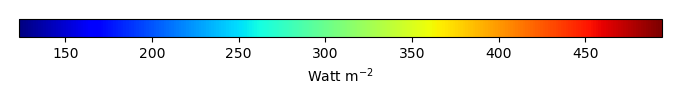MODEL MEANBIAS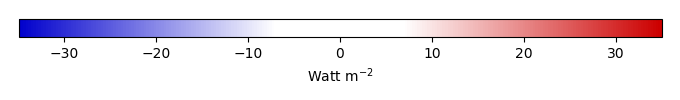BIAS SCORERMSE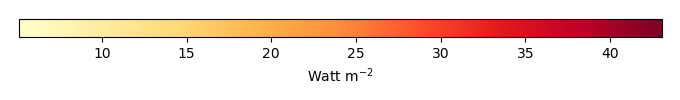RMSE SCOREBENCHMARK INTERANNUAL VARIABILITY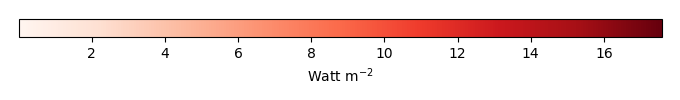MODEL INTERANNUAL VARIABILITYINTERANNUAL VARIABILITY SCOREBENCHMARK MAX MONTHMODEL MAX MONTHDIFFERENCE IN MAX MONTH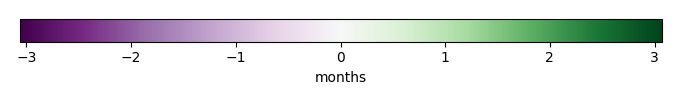SEASONAL CYCLE SCORESPATIAL TAYLOR DIAGRAMMODEL COLORS# Spatially integrated regional mean

MODEL COLORSREGIONAL MEANANNUAL CYCLEMONTHLY ANOMALYANNUAL CYCLE# All Models

BenchmarkCRUNCEPv7GSWP3v1WFDEI# Data Information

creation_date: Mon Jun 30 23:35:20 PDT 2014

source_file: This product is generated from monthly 1 degree GEWEX SRB Radiation observations